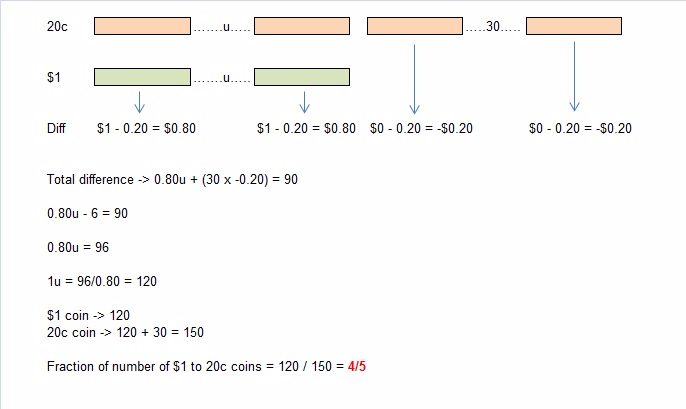# Question

30 x 0.20 = 6
90 + 6 = 96 (difference in value after removing 30 20c coins)
1 – 0.20 = 0.80 (difference
96/0.80 = 120 (number of \$1 coins)
120 + 30 = 150 (number of 20c coins)
120/150 = 4/5

Ans : 4/5.

0 Replies 0 Likes

30 coins of 20c = 30X 0.2 = \$6

If we remove 30 coins of 20c, they will be equal in number, but the difference between them will be \$96

For each \$1 and 20c, the difference is \$0.80
Therefore the number of \$1 coins = 96/0.8 = 120
Number of 20c coins = 120 + 30 = 150

Fraction of number of \$1 to 20c coins = 120/150 =>4/5 ###

1 Reply 1 Like

Even though the number of \$1 coin is less, but their total value is till \$90 more than the 20c coins.

Take a look at the answer, there are 120 of \$1 coins, the value is \$120.
There are 150 of 20c coins, value at \$30.
So if you remove 30 coins from 20c, to make them the same number as \$1 coins, which is 120 coins, the value of the 20c is = \$24. Which is a \$96 difference.

Basically I am saying, with 30 numbers of 20c coins is only \$6. \$6 or 30 more 20c coins is not going to be make the value of 20c to be \$90 more than total value of \$1 coins. Therefore we are correct to assume that the the value of \$1 coin is more than value of 20c coins even though there are 30 coins more with 20c. Removing the 20 numbers of 20c coins will only increase the difference further by \$6.

1 Reply 0 Likes

No apologies needed, glad you clear the doubt as it may help others. Many students will be wondering if the value of 20c coins will have more value or if the \$1 coins will have more value, and that makes a big difference if the student assumed wrongly that 20c coins is \$90 more than \$1 and proceed to calculate wrongly the difference as 90-6  = \$84.

You have given me the opportunity to explain why 30 coins of 20c will not make the value of the total 20c be more than value \$1 coins.

I like to add on a table to show this clearer,

 20c (+30 more than \$1) \$1 Difference in 20c – \$1 31 1 \$5.20 \$5.20 is the most a 30 extra number of 20c will be more than the value of \$1 coins 32 2 \$4.40 33 3 \$3.60 The difference get lesser 38 8 -\$0.40 So, no way an extra 30 number of 20c will ever be \$90 more than a \$1 coins, therefore it is the value of \$1 that is \$90 more than the value of 20c coins. Hence why we plus the difference instead of minus,
0 Replies 0 Likes1 Reply 0 Likes